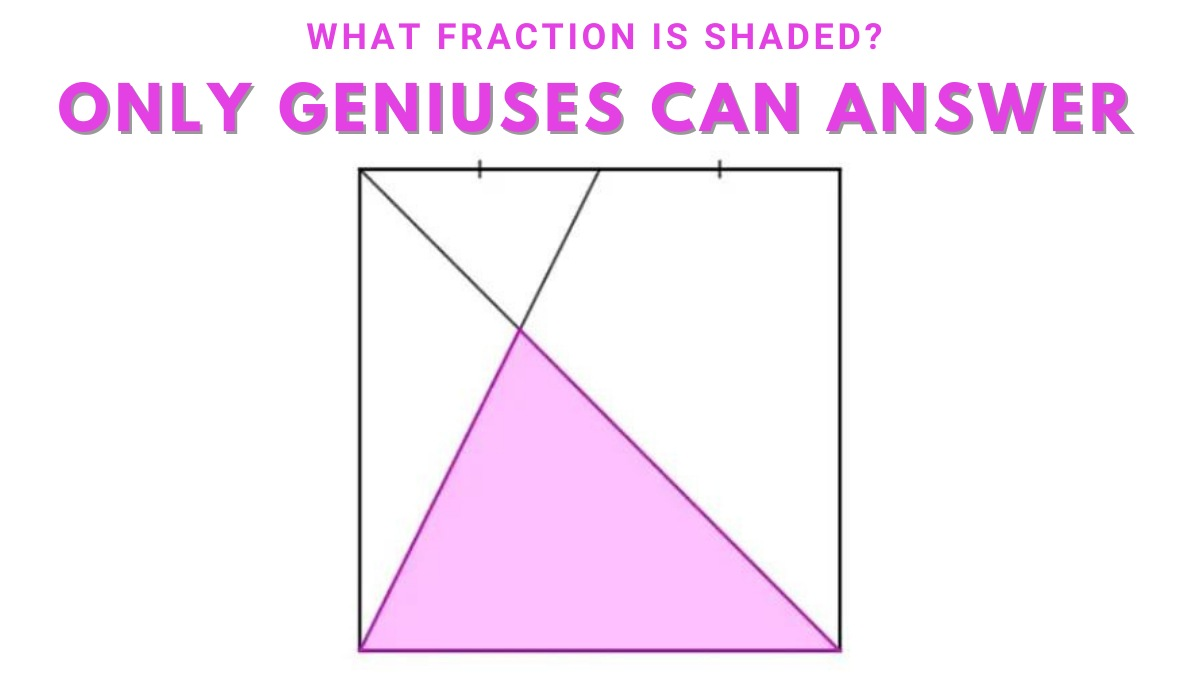# Math Riddle IQ Test: What Fraction Is Shaded? 99% Have Failed.

It has been proven that math riddles can make you smarter. But are you smart enough to solve this math riddle? Test yourself here.Math Riddle IQ Test: What Fraction Is Shaded? 99% Have Failed.

Are you looking for ways to improve your brain power? Math riddles are great for improving cognitive skills. They also boost creativity and problem-solving abilities.

Math puzzles are fun and challenging. They require you to solve problems using logic and reasoning. This helps develop your ability to focus, concentrate, and remember things.

There are many types of problems requiring arithmetic knowledge out there. Some are designed to test your knowledge of basic arithmetic. Others are more complex and require you to apply your logical thinking skills.

This is why we are back with another math riddle to help improve the functioning of the logical part of your brain.

## Math Riddle IQ Test: What Fraction is Shaded?

Look at the riddle posted below.

Math Riddles: 5 Questions On Calculating Area And Perimeter

Ed Southall posted this math riddle which is a picture of a pink triangle inside a square and asked viewers to estimate the fraction of the square that is pink.

If you are good at math, you can solve this riddle easily, but if math is not your strength then you will have to work hard to figure out the answer.

If you find this too easy, then we are turning this math riddle into a challenge for you.

You have to solve this in under 17 seconds.

All the best!

You must have figured out the answer by now.

If you have, congratulations are in order. We knew you could do this.

Still, we are going to reveal the answer so you can cross-check your calculations.

Presuming that the square is 1 x 1, we can now determine the base of the triangle to be 1 (as it is of the same length as the square). Now, we need to determine the height.

One important thing to remember is that the ratio of the triangles' heights to their bases will always be the same, which is a characteristic of identical triangles.

The original of the math riddle, Southall said, "The pink triangle's height is equal to that of the little triangle because its base is twice as large. However, since the pink triangle's height is 1/3 of the tiny triangle's height, we know that its height plus the little triangle's height equals 1. Connect it, and we obtain our area as 1/2 x base x height, or 1/2 x 1 x 2/3, or 1/3."

Hence, ⅓ fraction of the square is colored.

The answer has been confirmed to be correct by Ed Southall.

These 5 Math Riddles Are So Fun To Solve That Even Your Kids Will Love Them!

Get the latest General Knowledge and Current Affairs from all over India and world for all competitive exams.
खेलें हर किस्म के रोमांच से भरपूर गेम्स सिर्फ़ जागरण प्ले पर